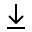MathBin Calculus Member

A calculus is the mathematical study of continuous change, in the same way that geometry is the study of shape and algebra is the study of generalizations of arithmetic operations. It has two major branches, differential calculus and integral calculus. Differential calculus concerns instantaneous rates of change and the slopes of curves. Integral calculus concerns accumulation of quantities and the areas under and between curves. These two branches are related to each other by the fundamental theorem of calculus. Both branches make use of the fundamental notions of convergence of infinite sequences and infinite series to a well-defined limit.

Bins in Two Dimensions